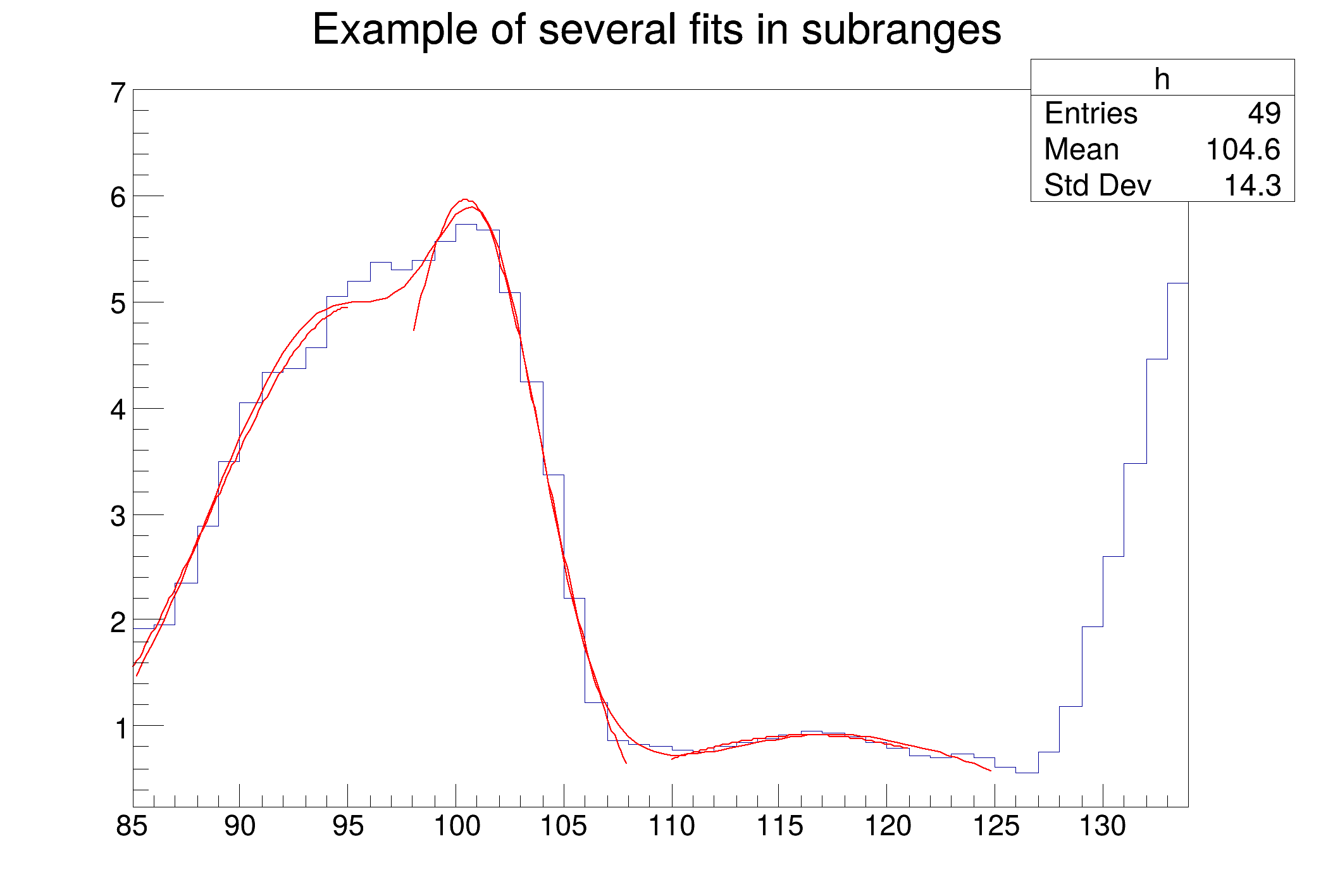ROOT   Reference Guidemultifit.C File Reference

## Detailed DescriptionFitting multiple functions to different ranges of a 1-D histogram Example showing how to fit in a sub-range of an histogram An histogram is created and filled with the bin contents and errors defined in the table below.

3 gaussians are fitted in sub-ranges of this histogram. A new function (a sum of 3 gaussians) is fitted on another subrange Note that when fitting simple functions, such as gaussians, the initial values of parameters are automatically computed by ROOT. In the more complicated case of the sum of 3 gaussians, the initial values of parameters must be given. In this particular case, the initial values are taken from the result of the individual fits.FCN=0.0848003 FROM MIGRAD STATUS=CONVERGED 105 CALLS 106 TOTAL
EDM=1.77382e-07 STRATEGY= 1 ERROR MATRIX ACCURATE
EXT PARAMETER STEP FIRST
NO. NAME VALUE ERROR SIZE DERIVATIVE
1 Constant 4.96664e+00 2.83221e+00 4.26889e-04 1.67619e-04
2 Mean 9.54663e+01 1.23905e+01 7.53972e-04 -2.63161e-04
3 Sigma 6.82779e+00 7.49131e+00 5.87496e-05 3.68521e-03
FCN=0.0771026 FROM MIGRAD STATUS=CONVERGED 72 CALLS 73 TOTAL
EDM=2.00364e-07 STRATEGY= 1 ERROR MATRIX ACCURATE
EXT PARAMETER STEP FIRST
NO. NAME VALUE ERROR SIZE DERIVATIVE
1 Constant 5.96312e+00 1.14355e+00 4.82019e-04 1.52951e-04
2 Mean 1.00467e+02 1.53372e+00 3.74926e-04 6.69980e-04
3 Sigma 3.54806e+00 1.16899e+00 3.22077e-05 3.86167e-03
FCN=0.0087702 FROM MIGRAD STATUS=CONVERGED 93 CALLS 94 TOTAL
EDM=5.57239e-07 STRATEGY= 1 ERROR MATRIX ACCURATE
EXT PARAMETER STEP FIRST
NO. NAME VALUE ERROR SIZE DERIVATIVE
1 Constant 9.12665e-01 4.37176e-01 1.46528e-04 2.91010e-04
2 Mean 1.16309e+02 8.37408e+00 3.57386e-03 -3.17966e-05
3 Sigma 8.38413e+00 1.84577e+01 4.99414e-04 -4.98793e-04
FCN=0.312817 FROM MIGRAD STATUS=CONVERGED 515 CALLS 516 TOTAL
EDM=1.73245e-07 STRATEGY= 1 ERROR MATRIX ACCURATE
EXT PARAMETER STEP FIRST
NO. NAME VALUE ERROR SIZE DERIVATIVE
1 p0 4.91145e+00 1.41387e+00 3.61239e-04 -3.22790e-04
2 p1 9.44525e+01 3.71612e+00 5.60861e-04 -6.78941e-05
3 p2 5.94796e+00 2.41732e+00 4.25396e-04 2.68176e-05
4 p3 3.22134e+00 3.11650e+00 5.86729e-04 -1.82620e-04
5 p4 1.01663e+02 1.67863e+00 5.56527e-04 3.95769e-04
6 p5 2.48454e+00 1.91461e+00 3.85832e-04 7.23818e-05
7 p6 9.11463e-01 3.68235e-01 1.45489e-04 5.77239e-04
8 p7 1.17582e+02 5.06329e+00 2.01798e-03 -8.25382e-05
9 p8 7.58627e+00 8.76000e+00 2.12468e-03 2.02614e-05
#include "TH1.h"
#include "TF1.h"
void multifit() {
const Int_t np = 49;
Float_t x[np] = {1.913521, 1.953769, 2.347435, 2.883654, 3.493567,
4.047560, 4.337210, 4.364347, 4.563004, 5.054247,
5.194183, 5.380521, 5.303213, 5.384578, 5.563983,
5.728500, 5.685752, 5.080029, 4.251809, 3.372246,
2.207432, 1.227541, 0.8597788,0.8220503,0.8046592,
0.7684097,0.7469761,0.8019787,0.8362375,0.8744895,
0.9143721,0.9462768,0.9285364,0.8954604,0.8410891,
0.7853871,0.7100883,0.6938808,0.7363682,0.7032954,
0.6029015,0.5600163,0.7477068,1.188785, 1.938228,
2.602717, 3.472962, 4.465014, 5.177035};
TH1F *h = new TH1F("h","Example of several fits in subranges",np,85,134);
h->SetMaximum(7);
for (int i=0;i<np;i++) {
h->SetBinContent(i+1,x[i]);
}
Double_t par;
TF1 *g1 = new TF1("g1","gaus",85,95);
TF1 *g2 = new TF1("g2","gaus",98,108);
TF1 *g3 = new TF1("g3","gaus",110,121);
TF1 *total = new TF1("total","gaus(0)+gaus(3)+gaus(6)",85,125);
total->SetLineColor(2);
h->Fit(g1,"R");
h->Fit(g2,"R+");
h->Fit(g3,"R+");
g1->GetParameters(&par);
g2->GetParameters(&par);
g3->GetParameters(&par);
total->SetParameters(par);
h->Fit(total,"R+");
}
#define h(i)
Definition: RSha256.hxx:106
int Int_t
Definition: RtypesCore.h:45
double Double_t
Definition: RtypesCore.h:59
float Float_t
Definition: RtypesCore.h:57
static unsigned int total
1-Dim function class
Definition: TF1.h:213
virtual Double_t * GetParameters() const
Definition: TF1.h:520
1-D histogram with a float per channel (see TH1 documentation)}
Definition: TH1.h:575
Double_t x[n]
Definition: legend1.C:17

Definition in file multifit.C.